# Maximum Product of Non-Negative Numbers 1

RN/01/14

W. B. Langdon W.Langdon@cs.ucl.ac.uk
Computer Science, University College, London,
Gower Street, London, WC1E 6BT, UK

14 January 2001

Suppose we wish to maximise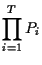(1)

subject to the conditions T>0,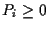,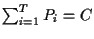and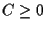.

Since logarithm is a monotonically increasing function for the range of P of interest we can maximise (1) by maximising its logarithm. We do this by differentiating w.r.t. Pi for 1<i<T subject to the constraints. The maximum value of (1) occurs when each of the partial derivatives is zero.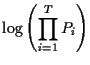=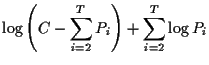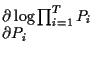=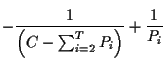Setting each partial derivative to zero (and noting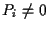) yields= 0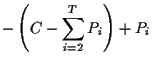= 0 -P1 + Pi = 0 Pi = P1

That is the Pi when (1) is maximal are all equal. Since they still have to sum to C every Pi =1/C.

W. B. Langdon
2001-01-14 (2 April 2001)